# Olympiad Test: Embedded Figures - 2

## 20 Questions MCQ Test National Cyber Olympiad Class 8 | Olympiad Test: Embedded Figures - 2

Description
Attempt Olympiad Test: Embedded Figures - 2 | 20 questions in 40 minutes | Mock test for Class 8 preparation | Free important questions MCQ to study National Cyber Olympiad Class 8 for Class 8 Exam | Download free PDF with solutions
QUESTION: 1

Solution:
QUESTION: 2

Solution:
QUESTION: 3

### Find out the alternative figure which contains figure (X) as its part.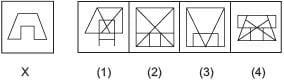Solution:
QUESTION: 4

Find out the alternative figure which contains figure (X) as its part.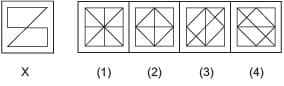Solution:
QUESTION: 5

Find out the alternative figure which contains figure (X) as its part.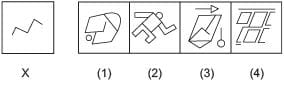Solution:
QUESTION: 6

Find out the alternative figure which contains figure (X) as its part.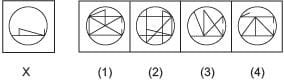Solution:
QUESTION: 7

Find out the alternative figure which contains figure (X) as its part.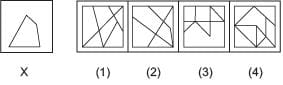Solution:
QUESTION: 8

Find out the alternative figure which contains figure (X) as its part.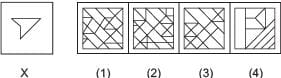Solution:
QUESTION: 9

Find out the alternative figure which contains figure (X) as its part.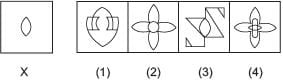Solution:
QUESTION: 10

Find out the alternative figure which contains figure (X) as its part.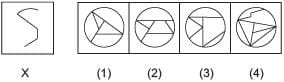Solution:
QUESTION: 11

Find out the alternative figure which contains figure (X) as its part.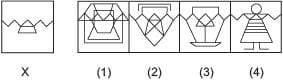Solution:
QUESTION: 12

Find out the alternative figure which contains figure (X) as its part.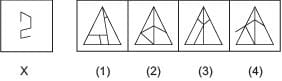Solution: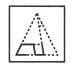option A is the correct answer

QUESTION: 13

Find out the alternative figure which contains figure (X) as its part.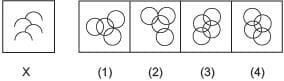Solution:
QUESTION: 14

Find out the alternative figure which contains figure (X) as its part.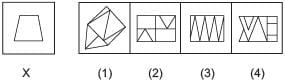Solution:
QUESTION: 15

Find out the alternative figure which contains figure (X) as its part.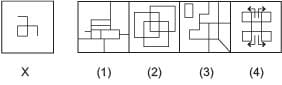Solution:
QUESTION: 16

Find out the alternative figure which contains figure (X) as its part.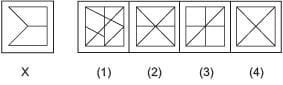Solution:
QUESTION: 17

Find out the alternative figure which contains figure (X) as its part.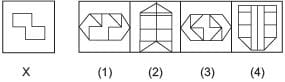Solution:
QUESTION: 18

Find out the alternative figure which contains figure (X) as its part.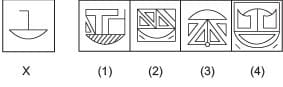Solution:
QUESTION: 19

Find out the alternative figure which contains figure (X) as its part.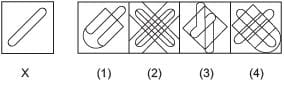Solution:
QUESTION: 20

An alloy is prepared by mixing three metals A, B and C in the proportion 3: 4: 7 by volume. Weights of the same volume of the metals A, B and C are in the ratio 5: 2: 6. In 130 kg of the alloy, the weight, in kg, of the metal C

Solution:

Required weight of C=(7×63×5+4×2+7×6)×130=84C=(7×63×5+4×2+7×6)×130=84 kgUse Code STAYHOME200 and get INR 200 additional OFF Use Coupon Code